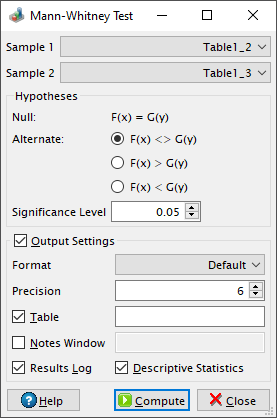# Mann-Whitney Test Dialog

This dialog is activated by selecting the Mann-Whitney Test... command from the Statistics -> Nonparametric Tests -> menu. It can be used in order to determine whether two independent samples were selected from populations having the same distribution. The Wikipedia article on the Mann-Whitney U test makes for excellent reading on this topic.

Figure 5-111. The two sample Mann-Whitney test dialog.Let us consider two independent samples of size `n1` and `n2` respectively. The test procedure includes the following steps:

1. Combine the two samples in a group.

2. Rank them in ascending order, beginning with 1 for the smallest value. Where there are groups of tied values, assign a rank equal to the average of unadjusted rankings.

3. Add up the ranks for the observations which came from the first sample (`R1`). The sum of ranks in the second sample, `R2`, can now be calculated, since the sum of all the ranks equals N(N+1)/2, `N` being the total number of observations: N = n1+n2.

4. Calculate U1 = R1-n1(n1+1)/2 and U2 = R2-n2(n2+1)/2. The test statistic `U` is the smaller value of `U1` and `U2`.

5. Calculate the approximate normal test statistic Z = [U-m-0.5(U-m)]/Var1/2, where 0.5 is a continuity correction factor, m = n1n2/2 is the mean and the variance Var = m(N+1)/6, in the absence of ties.

6. If there are ties in ranks, the following correction value is substracted from the variance: mT/[N(N-1)], where `T` can be calculated by summing the values tj(tj-1)(tj+1)/6 for each group of ties, `tj` being the number of ties in the j-th group.

7. Calculate a p-value for the approximate normal test statistic Z. The null hypothesis is rejected if the probability is lower than the value of the significance level.